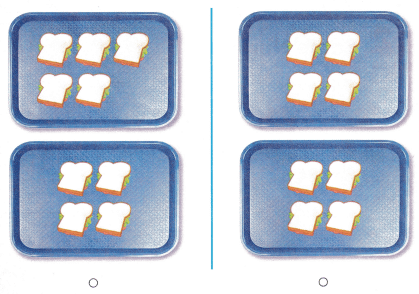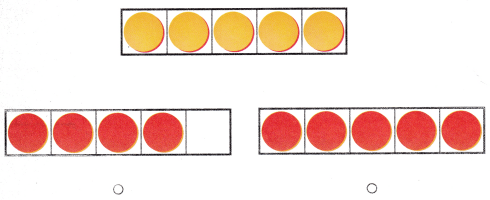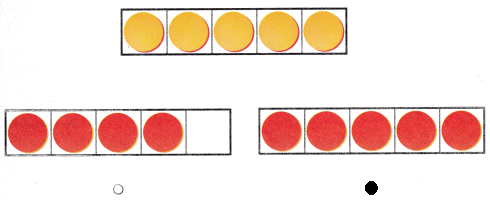Texas Go Math Kindergarten Lesson 3.2 Answer Key Same Number

Refer to our Texas Go Math Kindergarten Answer Key Pdf to score good marks in the exams. Test yourself by practicing the problems from Texas Go Math Kindergarten Lesson 3.2 Answer Key Same Number.

Texas Go Math Kindergarten Lesson 3.2 Answer Key Same Number

Explore

DIRECTIONS: Place counters as shown. Draw a line to match each counter in the top five frame to a counter below it in the bottom five frame. Count how many in each set. Tell a friend about the number of counters in each set.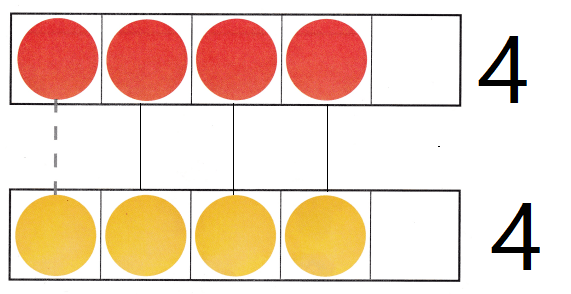Explanation:
Drawn the lines to match
and said a friend about the counters
and written the number

Share and Show

DIRECTIONS: 1. Place a counter on each car in the set as you count them. Move the counters to the five frame below the cars. Draw the counters. Place a counter on each finger puppet in the set as you count them. Move the counters to the five frame above the puppets. Draw those counters. Is the number of objects in one set the same or different than the number of objects in the other set? Draw lines to match the counters.

Question 1.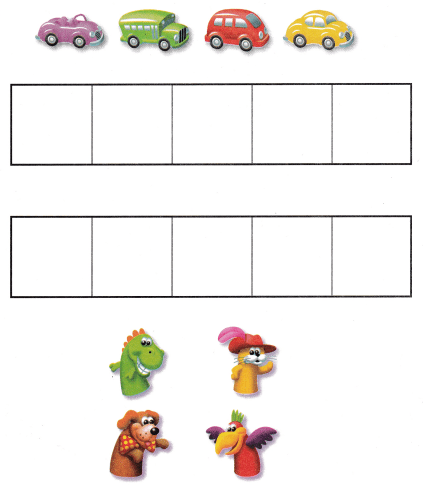Explanation:
The cars are marked with the counters
with the 5 frame
The puppets are handed with the counters
with the 5 frame
Matched the first frame and the second frame
both the frame has 4 counters

DIRECTIONS: 2. Write the number of hats. Write the number of juice boxes. Is the number of hats the same or different than the number of juice boxes?

Question 2.Explanation:
Both the sets have 5 each
Number of hats is equal to number of juices.

HOME ACTIVITY • Show your child two sets that have the same number of up to five objects. Have him or her identify whether the number of objects in one set is the same or different than the number of objects in the other set.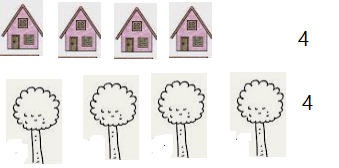Explanation:
The objects in the two sets are same
Counted and written the number.

DIRECTIONS: 3. Write the number of buses. Draw to show a set of counters that has the same number as the set of buses. Write the number. Draw a line to match the objects in each set. 4. Choose the correct answer. Which shows the same number?

Problem Solving

Question 3.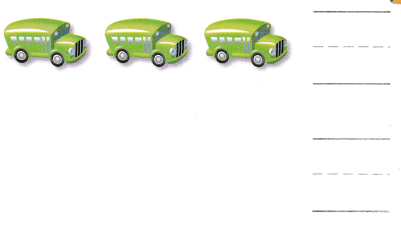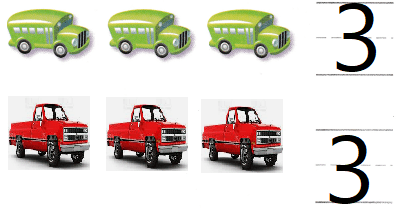Explanation:
There are 3 buses to equal them three  jeeps are drawn
And written the counters

Question 4.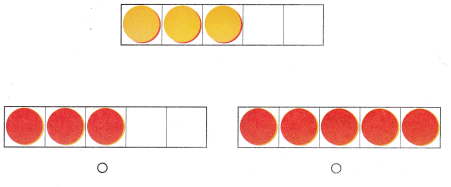Explanation:
There are 3 yellow circles
matched with the first set
and bubbled the correct option.

Texas Go Math Kindergarten Lesson 3.2 Homework and Practice Answer Key

DIRECTIONS: 1. Write the number of flowers. Write the number of orange leaves. Is the number of flowers the same or different than the number of orange leaves?

Question 1.Explanation:
The set of flowers are equal to set of leaves
Written the numbers

DIRECTIONS: Choose the correct answer. 2. Which shows the same number of sandwiches? 3. Which shows the same number?

Lesson Check

Question 2.